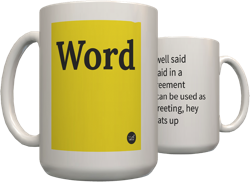Top definition
The format for a number written in base-16 instead of base-10 (decimal). Commonly used in computing or electronic fields, since each digit in a hexadecimal number is trivially converted (by humans or machines) into 4 bits with a table. A number in base-10 is more difficult to convert into binary, requiring repeated division by 2.

When writing a hexadecimal number, for digits greater than '9' convention dictates use of the first 6 letters of the alphabet. So the digits are:
0 1 2 3 4 5 6 7 8 9 A B C D E F
The value of the popular magic number DEADBEEF when converted from hexadecimal to decimal is 3735928559.
by pr0ntab January 08, 2004